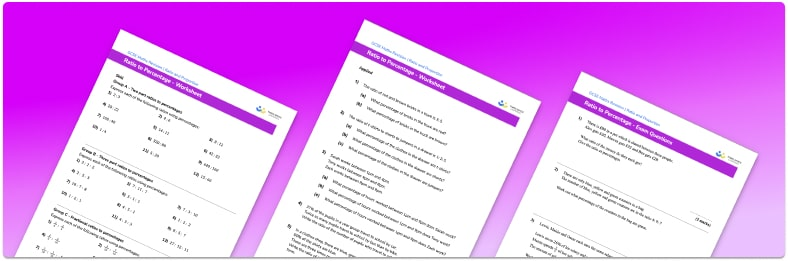# Ratio To Percentage Worksheet• Section 1 of the ratio to percentage worksheet contains 20+ skills-based ratio to percentage  questions, in 3 groups to support differentiation
• Section 2 contains 3 applied ratio to percent problems with a mix of worded problems and deeper problem solving questions
• Section 3 contains 3 foundation and higher level GCSE exam style ratio to percentage questions
• Answer keys and a mark scheme for all practice ratio to percentage questions are provided
• Questions follow variation theory with plenty of opportunities for students to work independently at their own level
• All questions created by fully qualified expert secondary maths teachers
• Suitable for GCSE maths revision for AQA, OCR and Edexcel exam boards

• This field is for validation purposes and should be left unchanged.

You can unsubscribe at any time (each email we send will contain an easy way to unsubscribe). To find out more about how we use your data, see our privacy policy.

### Ratio to percentage at a glance

We can take the information in a given ratio and use it to write the percentage that each part of the ratio represents. For example, if the ratio of the number of boys to the number of girls in a class is 2:3 then we can see that 2 fifths of the students are boys and 3 fifths are girls. 2 fifths is equal to 40% and 3 fifths is equal to 60%. Therefore 40% of the students are boys and 60% are girls. A percentage must have a percent sign.

The same idea can be applied to 3 part ratios and ratios with any number of parts. For example if the ratio of yellow counters:red counters:blue counters in a bag is 2:3:5 then the fractions would be frac{2}{10} : frac{3}{10} : frac{5}{10} and the percentages would be 20% : 30% : 50%.

Looking forward, students can then progress to additional ratio worksheets and other ratio and proportion worksheets, for example a ratio worksheet or a simplifying ratios worksheet.For more teaching and learning support on Ratio and Proportion our GCSE maths lessons provide step by step support for all GCSE maths concepts.

## Do you have KS4 students who need more focused attention to succeed at GCSE?There will be students in your class who require individual attention to help them succeed in their maths GCSEs. In a class of 30, it’s not always easy to provide.

Help your students feel confident with exam-style questions and the strategies they’ll need to answer them correctly with our dedicated GCSE maths revision programme.

Lessons are selected to provide support where each student needs it most, and specially-trained GCSE maths tutors adapt the pitch and pace of each lesson. This ensures a personalised revision programme that raises grades and boosts confidence.

Find out more